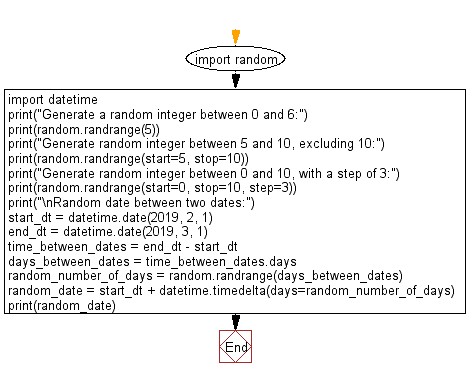﻿ Python: Generate a random integer and date - w3resource# Python: Generate a random integer and date

## Python module: Exercise-5 with Solution

Write a Python program to generate a random integer between 0 and 6 - excluding 6, random integer between 5 and 10 - excluding 10, random integer between 0 and 10, with a step of 3 and random date between two dates.

Use random.randrange()

Sample Solution:

Python Code:

``````import random
import datetime
print("Generate a random integer between 0 and 6:")
print(random.randrange(5))
print("Generate random integer between 5 and 10, excluding 10:")
print(random.randrange(start=5, stop=10))
print("Generate random integer between 0 and 10, with a step of 3:")
print(random.randrange(start=0, stop=10, step=3))
print("\nRandom date between two dates:")
start_dt = datetime.date(2019, 2, 1)
end_dt = datetime.date(2019, 3, 1)
time_between_dates = end_dt - start_dt
days_between_dates = time_between_dates.days
random_number_of_days = random.randrange(days_between_dates)
random_date = start_dt + datetime.timedelta(days=random_number_of_days)
print(random_date)
```
```

Sample Output:

```Generate a random integer between 0 and 6:
0
Generate random integer between 5 and 10, excluding 10:
5
Generate random integer between 0 and 10, with a step of 3:
3

Random date between two dates:
2019-02-17
```

Flowchart:## Visualize Python code execution:

The following tool visualize what the computer is doing step-by-step as it executes the said program:

Python Code Editor:

Have another way to solve this solution? Contribute your code (and comments) through Disqus.

What is the difficulty level of this exercise?

Test your Python skills with w3resource's quiz

﻿

## Python: Tips of the Day

For-else construct useful when searched for something and find it:

```# For example assume that I need to search through a list and process each item until a flag item is found and
# then stop processing. If the flag item is missing then an exception needs to be raised.

for i in mylist:
if i == theflag:
break
process(i)
else:
raise ValueError("List argument missing terminal flag.")
```